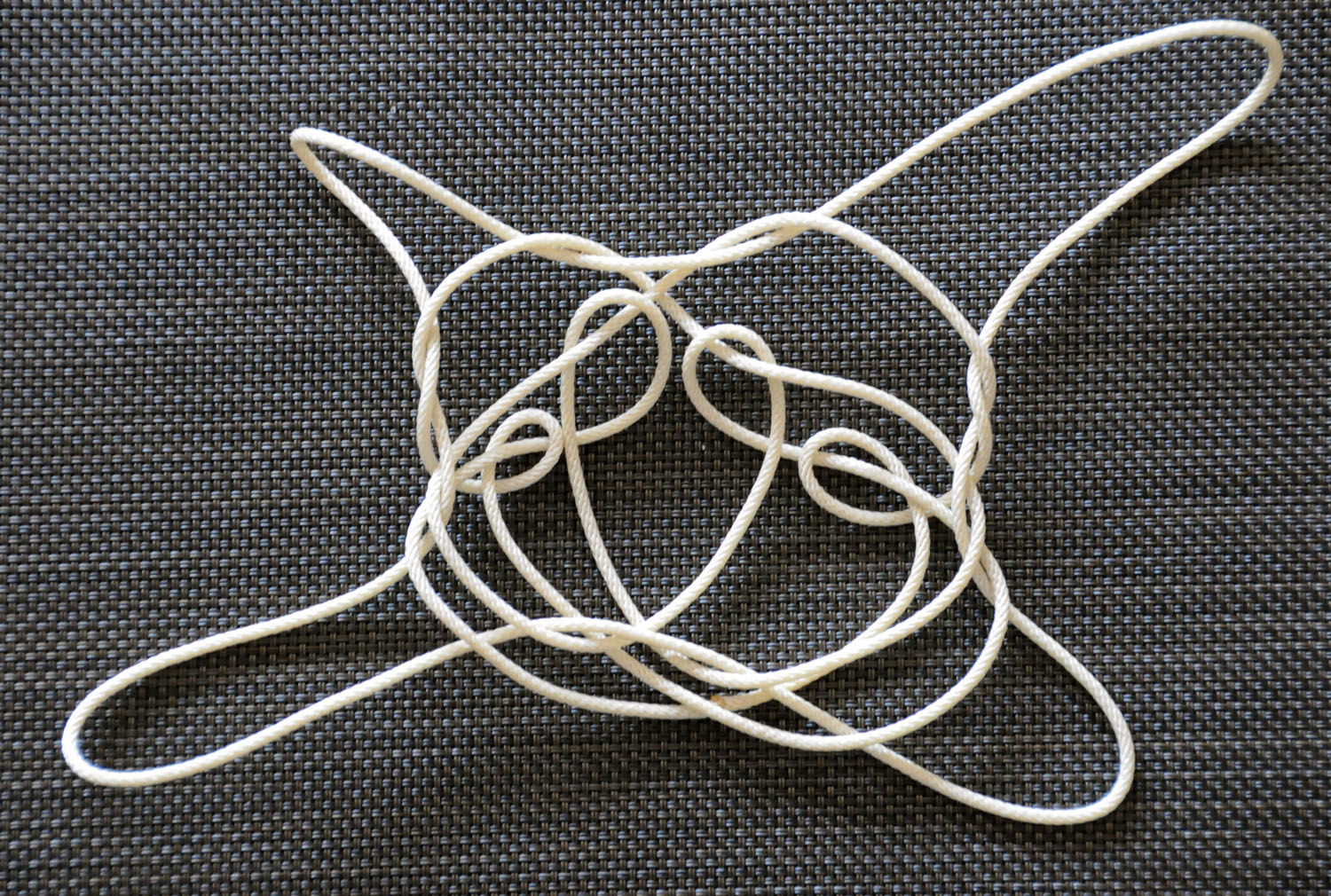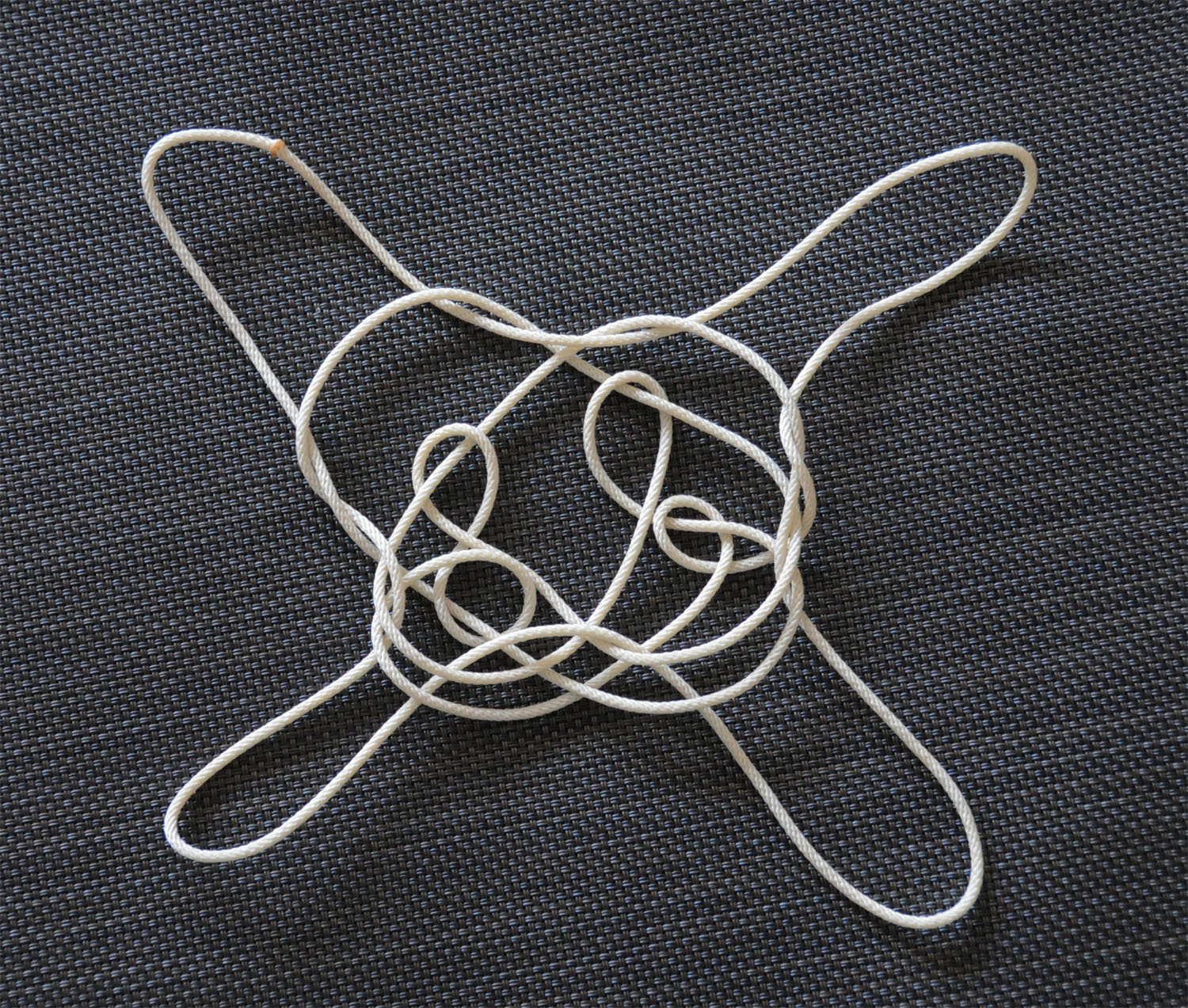# 4 loop dominant switches and dominant transfers

these videos sketch in the matrix of possibilities for forming 4 loop figures with two operations on each interior loop.

4 loop openings with dominant switches and dominant transfers

the eight 4 loop openings to be considered are:

picking up the near string from the little fingers with the left hand first by curling the left thumb down into little finger loop near the right hand to pick up the near little finger string and beginning to return left hand to position but then introducing right thumb from below into loop forming on thumbs and returning hands to position. this forms two loops on the hands, thumbs and little fingers. transfer the thumb loop to the index finger by inserting index fingers into thumb looops from below. and, then again picking up the near transverse with the left hand first as above and transferring resultant index loops to middle fingers and thumb loops to index fingers. and then again picking up the near transverse with the left hand first as above so that the resulting four loops are on the thumbs, index, middle, and little fingers.

this will be referred to with the short hand code l l l and is what i consider to be 4 loop dna opening. then in similar fashion all eight possibilities can be achieved:

l l l
l l r
l r l
l r r

r r r
r r l
r l r
r l l

note the division into fours indicated the chiral symmetry of the openings.

care should be taken at this point to remind oneself that the loops on the hands should be “read” in pairs to understand what happens when dominant switches and dominant transfers are performed on the index and middle finger loops.

and one should remind oneself that the four 3 loop openings are as follows:

l l (ldna)
l r (opening B)
r l (opening A)
rr (rdna)

this means that in the case of the l r l opening the middle finger loops are formed by the l r pair (one must be sure to understand that the pair should be read right to left as they were performed!!) and thus can be considered the equivalent of an opening B, and the index loops are formed by the r l pair and can be considered to be the equivalent of an opening A.

we already know that opening B forms the inuit hole with RS or right dominant switch moves and that opening A forms the inuit hole with LS or left dominant switch moves. and for the prototypical test pattern of this photo essay the index loops would be manipulated with two successive LS moves and the middle fingers with two successive RS moves. then the switching of the loops to be ready for the inuit ending should be performed. then after this ending the following is the result:to introduce the sort of differences i am interested in, contrast the picture above with the picture for the l l l 4 loop opening followed by two successive LS moves on the index loops and then two successive LS moves on the middle finger loops. the image is below.note the two loops on the right side of the image are the same, but the left pair are considerably different in their formation.

next comes the l l r opening followed by two successive RS moves on the index loops and two successive LS moves on the middle finger loops.

note! this is because the appropriate inuit hole formations occur even though the l l pair on the index “should?” use the 2LS.

the following results:and the fourth figure is from the l r r opening with two successive RS moves on the index finger loops and two successive RS moves on the middle finger loops.

note again that one “should?” expect 2LS moves on the index loops.

the following results:then in summary

the first figure (l r l 2LS 2LS) has two figure eights
the second figure (l l l 2LS 2LS) has a figure eight on the right
the third figure (l l r 2RS 2LS) has no figure eight
the fourth figure (l r r 2RS 2RS) has a figfure eight on the left

this is not what i expected, but it is what i produced when i formed the figures. i fully expect the four openings r r r, r r l, r l r, and r l l will demonstrate the four different patterns when formed with the appropriate dominant switches.the first figure (r r l 2LS 2RS) has no figure eightsthe second figure (r r r 2RS 2RS) has a figure eight on the rightthe third figure (r l r 2RS 2LS) has two figure eightsthe fourth figure (r l l 2LS 2LS) has no figure eights